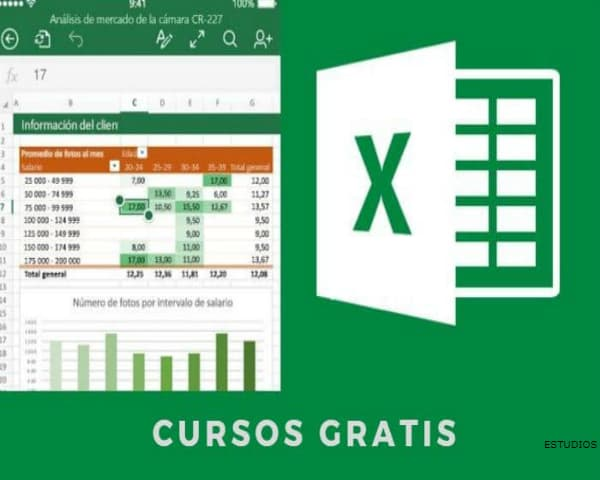Saltar al contenido# FREE ALGEBRA COURSE

Algebra is an area of mathematics that uses symbols to represent numbers in formulas and equations.Â Understanding these symbols and how they work together to provide structure to equations allows mathematicians to write formulas more efficiently and to solve mathematical problems.

Today there are several universities, institutions and educational centers, among others, that provide free courses or classes to help or reinforce knowledge and skills in the area of Mathematics, Algebra and Geometry.

So if you are interested in acquiring knowledge or improving your skills in algebra it is a good time to take a free course. These courses help your participant to build a solid foundation in these areas.Â You can also find free online Algebra courses, with online quizzes and algebra worksheets, whose mission is to provide a free quality education to anyone, anywhere. All you need is an Internet connection and the willingness to learn.

## What is a Algebra Course ?

An Algebra Course is an educational program taught by a university, institution or educational center, which is designed to provide or reinforce knowledge and improve skills in the area of mathematics and even more emphasized in algebra and trigonometry.

These courses are designed with appropriate didactics to make algebra learning a student’s subject matter.Â The Algebra Courses possess critical thinking through the resolution of equations and the understanding of algebraic expressions.

The courses will rely heavily on elements that include quadratic formulas, systems of equations, polynomial expressions, rational expressions, rational functions, quadratic equations, polynomial functions, algebraic operations, graphs, square roots, complex numbers, and more.

Algebra is essential to the high school and college math curriculum and these Free Algebra Courses will serve as a pre-algebra course. Working through these algebra problems will prepare you well for future math study.

## Algebra Course Program, Subjects and Content

These are some of the areas covered in the program content of an Algebra Course:

• Discovering expressions, equations and functions
• Exploring real numbers
• Linear functions
• How to solve linear equations
• Formulation of linear equations
• Linear Inequalities
• Systems of linear equations and inequalities
• How to solve a system of linear equations
• Graphing Linear Functions and Equations
• Exponents and exponential functions.
• Factorization and polynomials
• Equations and inequalities.
• Matrices
• Conical Sections
• Polynomial functions
• Rational expressions
• Exponential and logarithmic functionsSequences and series
• Discrete mathematics and probability
• Trigonometry

## Countries and Cities Where You Could Study the Algebra Course for Free Getting a Scholarship

• United States
• United Kingdom
• Mexico
• Australia
• Spain

## Institutes, Centers and Universities Where the Algebra Course is Possibly Free, through Scholarships

• Math Planet
• Online.

• Math Planet
• Online.

### Free Introduction to Algebra Course

• Edx – SchoolYourself
• Online.

• Edx – ASUx

### Online.Free Intermediate Algebra Course

• University of California, Irvine
• Via Coursera
• Online.

### Free Introduction to Algebra Course

• The Open University – Open Learn
• Online.

### Free Pre-Calculus Algebra Course

• Through Canvas Network
• Online.

### Free Pre-Calculus Algebra Course

• University of California, Irvine
• Through Coursera
• Online.

### Free Course Visualizing Algebra

• San Jose State University
• Through Udacity
• Online.

### Free University Algebra Course

• San Jose State University
• Through Udacity
• Online.

## Click for Other Studies at Different Academic Levels

Related Topics

Other Topics of Interest in ALPHAPEDIA

## Image of Free CourseResumen / SummaryTÃ­tulo / Article Name
FREE ALGEBRA COURSE
DescripciÃ³n / Description
Free Algebra Course: What It Is, Its Objective, Content and Subjects, Universities, Centers, Countries and Cities Where it is Offered
Autor / Author
Autor / Publisher Name
ALPHAPEDIA
Logo / Publisher Logo